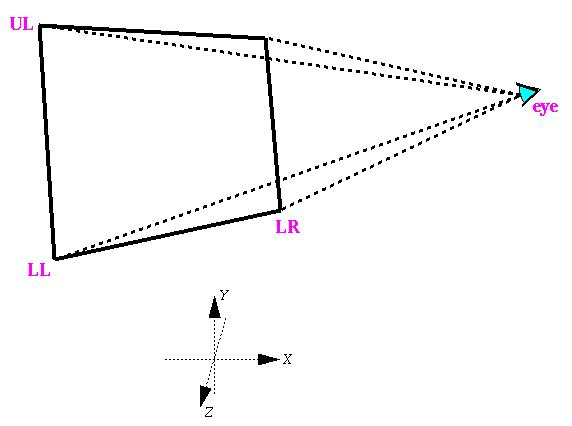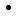# Computing the CAVE Projection Transformation

Dave Pape, pape@evl.uic.edu

This report describes the steps involved in computing a geometrically accurate projection transformation for a CAVE, ImmersaDesk, or IWall VR display (hereafter referred to simply as a CAVE). The CAVE projection assumes a fixed rectangular display screen that can be at any arbitrary position in space, with the viewer's eye able to move anywhere in front of the screen; see figure 1. To compute the projection, we are given the positions of the corners of the screen - LL (lower left), UL (upper left), and LR (lower right) - and the current tracked position of the viewer's eye. (Note: since the screen is known to be a rectangle, only three corner positions are needed.)Figure 1. CAVE screen and eye-point in CAVE space

As the eye-point can be anywhere relative to the screen, the viewing volume is, in general, an off-axis frustum. In OpenGL, we create the projection matrix using glFrustum(). The glFrustum() matrix assumes an eye-point at the origin, looking down the negative Z axis, with the projection plane parallel to the X-Y plane (figure 2); hence, to complete the projection, we must also compute a matrix which transforms the screen and eye-point from the situation of figure 1 to that of figure 2. This second transformation is loaded as the View matrix in OpenGL.Figure 2. glFrustum() off-axis viewing volume (screen space)

The "real-world" coordinate system of figure 1 will be referred to as CAVE space; the projection coordinate system of figure 2 will be referred to as screen space.

## Screen-space axes and transformation

First, given the positions of the screen corners, we compute the coordinate axes of screen-space (Xs, Ys, Zs), in CAVE coordinates. The X axis corresponds to the horizontal edge of the screen, pointing to the right. The Y axis is the vertical edge of the screen, pointing up. The Z axis is then the cross product of X and Y. We also compute the width and height of the screen at this time, as these values will be needed in determining the frustum.
```	right = LR - LL

width = || right ||

Xs = right / width

up = UL - LL

height = || up ||

Ys = up / height

Zs = Xs x Ys
```
Given these axes, we can compute the rotation portion of the view matrix for the CAVE-to-screen-space transformation. Since (Xs,Ys,Zs) are the screen-space coordinate axes in CAVE-space, they define a transformation from screen- to CAVE-space:
```	| Xs  Ys  Zs |
| Xs  Ys  Zs |
| Xs  Ys  Zs |
```
The desired transformation is then just the inverse of this matrix.
```		| Xs  Ys  Zs | -1
RotMat=	| Xs  Ys  Zs |
| Xs  Ys  Zs |
```

## Off-axis frustum

To create the projection matrix with glFrustum(), we must determine the distances to the six clipping planes - left, right, bottom, top, near, and far. In the CAVE system, the near and far clipping distances are defined by the application program; the remaining values are calculated from the tracked eye-point and the previously computed screen coordinate axes. We first calculate the values as absolute distances on the plane of the screen itself (L, R, B, T); they will then be scaled appropriately for glFrustum().

As shown in figure 2, the value of L is the distance from the eye-point to the left edge of the screen, along the Xs axis. Similarly, B is the distance from the eye-point to the bottom edge of the screen, along the Ys axis. Therefore, these values can be computed by taking the dot product of the screen axes with the eye position relative to the lower-left screen corner. R and T can then be computed using the width and height of the screen.

```	eyes = eye - LL

L = eyesXs

R = width - L

B = eyesYs

T = height - B
```
The left/right/bottom/top arguments for glFrustum() must define the corners of the near clipping plane. Using similar triangles, we compute these values from L/R/B/T, scaling them by the ratio of the near clipping distance to the distance between the eye-point and the screen:
```	distance = eyesZs

left =   -L * near / distance

right =   R * near / distance

bottom = -B * near / distance

top =     T * near / distance
```

## View transformation

The view matrix transformation, which transforms from the CAVE-space of figure 1 to the screen-space of figure 2, consists of two parts - a rotation to orient the projection plane parallel to the X/Y plane, and a translation to put the eye-point at the origin. The rotation part was compute above, from the screen-space coordinate axes. The translation is merely the negation of the eye position (in CAVE space):
```	ViewMat = translate(-eye,-eye,-eye) * RotMat
```